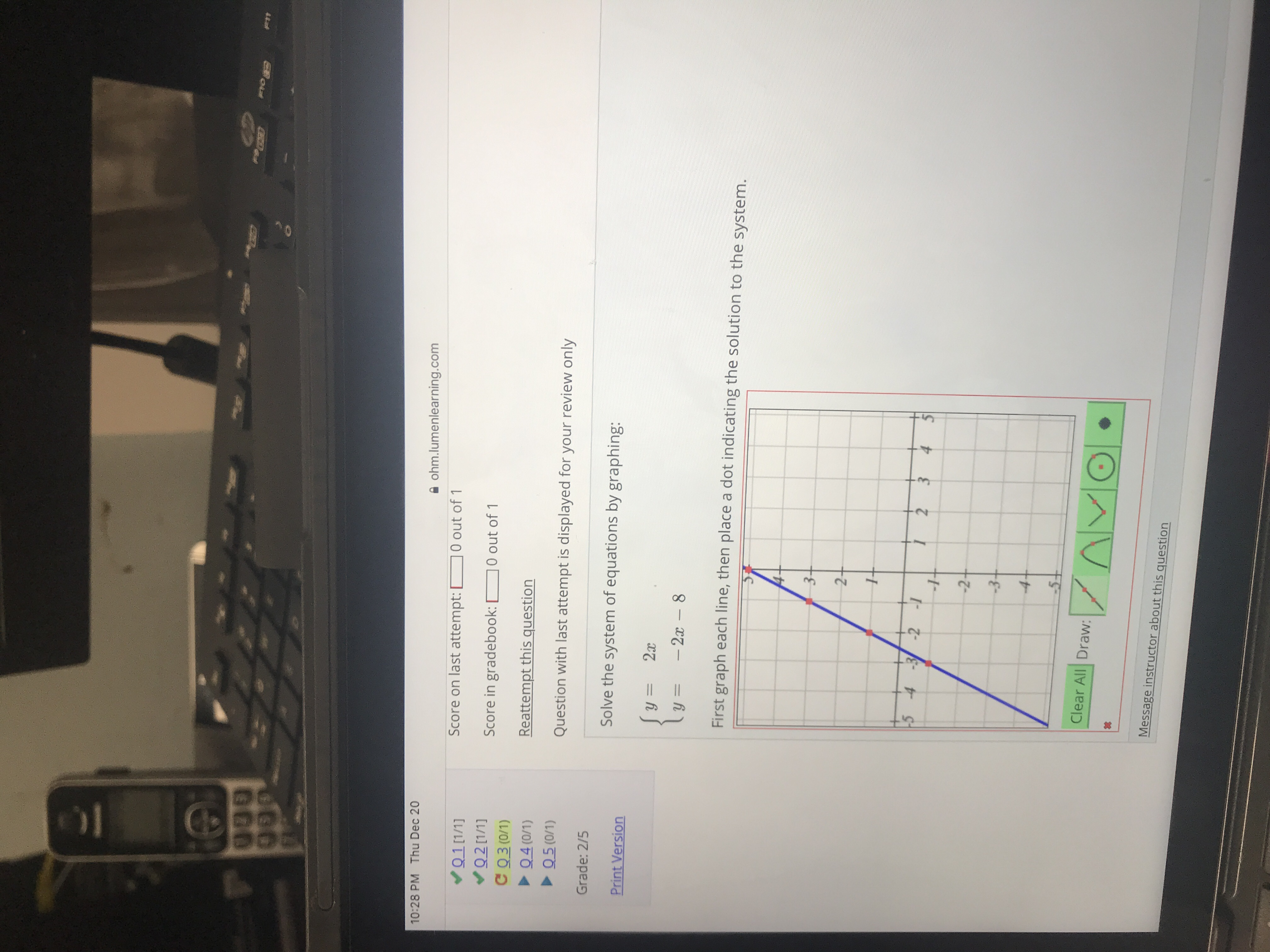# 10:28 PM Thu Dec 20ohm.lumenlearning.comScore on last attempt:0 out of 1Score in gradebook: 0 out of 1Reattempt this questionС Q30/1)04 (0/1)uestion with last attempt is displayed for your review onlyGrade: 2/5Solve the system of equations by graphing:Print Vy2First graph each line, then place a dot indicating the solution to the system2Clear All Draw:Message instructor about this question

Question
3 viewshelp_outlineImage Transcriptionclose10:28 PM Thu Dec 20 ohm.lumenlearning.com Score on last attempt:0 out of 1 Score in gradebook: 0 out of 1 Reattempt this question С Q30/1) 04 (0/1) uestion with last attempt is displayed for your review only Grade: 2/5 Solve the system of equations by graphing: Print V y2 First graph each line, then place a dot indicating the solution to the system 2 Clear All Draw: Message instructor about this question fullscreen
check_circle

Step 1

Here two equations are given, we need to find the solution to the system of equations by plotting them.

Step 2

Plotting the line y = 2x .. To plot a straight line we need to determine any two points on the line.

Let us substitute x= 1 and 2 in the line equation and see their respective y values. So that we get two points on the given line.

After substituting x = 1 we get y = 2 and for x = 2 we get y = 4. So the points on the straight line are (1,2) and (2,4).

Step 3

To plot any grpah we need an scale, we assume one unit =1.

Here we can see the points (1,2) and (2,4) are...

### Want to see the full answer?

See Solution

#### Want to see this answer and more?

Solutions are written by subject experts who are available 24/7. Questions are typically answered within 1 hour.*

See Solution
*Response times may vary by subject and question.
Tagged in

### Algebra